﻿ Adding an Unbound Row to a Bound Sheet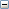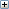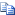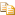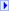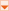Spread Windows Forms 6.0 Product Documentation Adding an Unbound Row to a Bound Sheet Support Options
 Spread Windows Forms 6.0 Product Documentation > Developer's Guide > Managing Data Binding > Adding to Bound Data > Adding an Unbound Row to a Bound Sheet

Glossary Item Box

Once you bind a sheet to a data set you might want to add an unbound row to contain additional data.

The following figure shows a sheet in a Spread component that contains data from a data set and an unbound row at the bottom that calculates the averages.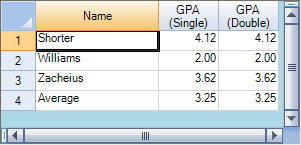#### Using a Shortcut

2. Set the FpSpread or Sheet DataSource property equal to the data set.
3. Call the Sheet AddUnboundRows method to specify where to add the unbound row.
4. Set properties for the unbound row.

#### Example

This example code binds the Spread component to a data set then adds an unbound row.

C#Copy Code
```// Bind the component to the data set.

```
VBCopy Code
```' Bind the component to the data set.

```

#### Using Code

2. Create a new SheetView object.
3. Set the SheetView object DataSource property equal to the data set.
4. Call the SheetView object AddUnboundRows method to specify where to add the unbound row.
5. Set properties for the unbound row.
6. Assign the SheetView object to a sheet in the component.

#### Example

This example code creates a bound SheetView object and adds an unbound row to it, then assigns it to a sheet in a Spread component.

C#Copy Code
```// Create a new SheetView object.

// Bind the SheetView object to the data set.

newsheet.DataSource = dataSet1;

// Assign the SheetView object to the first sheet.

```
VBCopy Code
```' Create a new SheetView object.

' Bind the SheetView object to the data set.

newsheet.DataSource = DataSet1